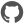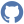# CompositeVector

Namespace: GibbsSampling

Consists of functions to work with Frequency- and Probability-Composite-Vectors.

## Nested types and modules

 Type Description FrequencyCompositeVector One dimensional array with fixed positions for each element. Use to track frequency of elements independent of position in source. ProbabilityCompositeVector

### Functions and values

 Function or value Description ``` calculateSegmentScoreBy pcv bioItems ``` Signature: pcv:ProbabilityCompositeVector -> bioItems:(type) -> float Type parameters: '?7928Calculate the score of the given sequence based on the probabilities of the ProbabilityCompositeVector. ``` createFCVOf resSources ``` Signature: resSources:(type) -> FrequencyCompositeVector Type parameters: '?7909Create a FrequencyCompositeVector based on BioArrays and exclude the specified segments. ``` createFCVWithout (...) ``` Signature: motiveLength:int -> position:int -> resSource:(type) -> FrequencyCompositeVector Type parameters: '?7913Create FrequencyCompositeVector based on BioArray and excludes the specified segment. ``` createNormalizedPCVOfFCV (...) ``` Signature: alphabet:'?7926 [] -> pseudoCount:float -> frequencyCompositeVector:FrequencyCompositeVector -> ProbabilityCompositeVector Type parameters: '?7926Create normalized ProbabilityCompositeVector based on FrequencyCompositeVector. ``` createPCVOf caArray ``` Signature: caArray:FrequencyCompositeVector -> ProbabilityCompositeVectorCreate a ProbabilityCompositeVector based on a FrequencyCompositeVector by replacing the integers with floats. ``` fuseFrequencyVectors alphabet bfVectors ``` Signature: alphabet:'?7911 [] -> bfVectors:FrequencyCompositeVector [] -> FrequencyCompositeVector Type parameters: '?7911Create new FrequencyCompositeVector based on the sum of the positions of an array of FrequencyVectors. ``` increaseInPlaceFCV (...) ``` Signature: bioItem:'?7905 -> frequencyCompositeVector:FrequencyCompositeVector -> FrequencyCompositeVector Type parameters: '?7905Increase counter of position by 1. ``` increaseInPlaceFCVBy (...) ``` Signature: bioItem:'a -> frequencyCompositeVector:FrequencyCompositeVector -> n:int -> FrequencyCompositeVector Type parameters: 'aIncrease counter of position by n. ``` increaseInPlaceFCVOf (...) ``` Signature: resSources:(type) -> backGroundCounts:FrequencyCompositeVector -> FrequencyCompositeVector Type parameters: '?7915Create FrequencyCompositeVector based on BioArray. ``` increaseInPlacePCV (...) ``` Signature: bioItem:'a -> backGroundProbabilityVector:ProbabilityCompositeVector -> ProbabilityCompositeVector Type parameters: 'aIncrease counter of position by 1. ``` increaseInPlacePCVBy (...) ``` Signature: bioItem:'a -> n:float -> backGroundProbabilityVector:ProbabilityCompositeVector -> ProbabilityCompositeVector Type parameters: 'aIncrease counter of position by n. ``` substractSegmentCountsFrom (...) ``` Signature: source:(type) -> fcVector:FrequencyCompositeVector -> FrequencyCompositeVector Type parameters: '?7917Subtracts the amount of elements in the given source from the FrequencyCompositeVector.